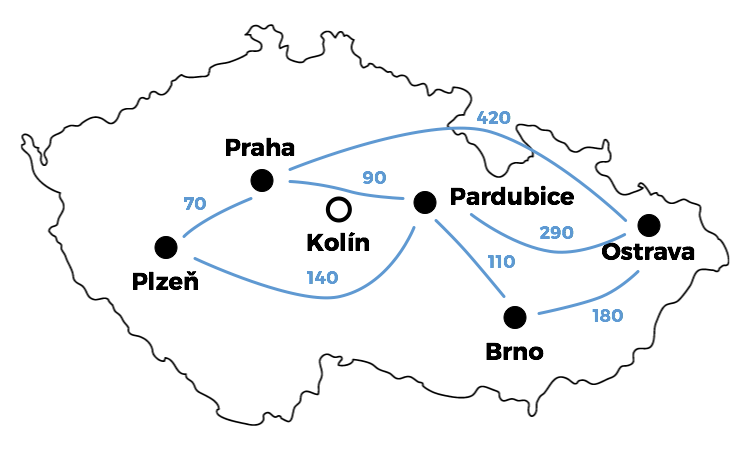## SwiftyDijkstra

### master

Simple objective implementation of Dijkstra algorithm (shortest path search) written in Swift

# SwiftyDijkstra

Example Dijkstra algorithm (shortest path problem) implementation written in Swift.

## Usage```import Foundation
import SwiftyDijkstra

// Define the nodes

let Brno = Node("Brno")
let Praha = Node("Praha")
let Ostrava = Node("Ostrava")
let Pardubice = Node("Pardubice")
let Kolin = Node("Kolin")
let Plzen = Node("Plzen")

// Define the graph edges

var edges = [
Edge(from: Ostrava, to: Brno, cost: 180),
Edge(from: Praha, to: Ostrava, cost: 420),
Edge(from: Pardubice, to: Brno, cost: 110),
Edge(from: Pardubice, to: Praha, cost: 90),
Edge(from: Pardubice, to: Plzen, cost: 140),
Edge(from: Pardubice, to: Ostrava, cost: 290),
Edge(from: Plzen, to: Praha, cost: 70),
]

// Initialize the graph itself

var G = Graph(edges: edges)

// Look for shortest paths and print'em

print(G.shortestPath(from: Praha, to: Ostrava))
// outputs [Praha, Pardubice, Ostrava]
print(G.shortestPath(from: Ostrava, to: Plzen))
// outputs [Ostrava, Pardubice, Plzen]
print(G.shortestPath(from: Ostrava, to: Ostrava))
// outputs [Ostrava]
print(G.shortestPath(from: Brno, to: Kolin))
// outputs []
```